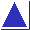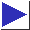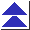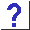# comp.lang.c FAQ list ·Question 20.21

Q: There seem to be a few missing operators, like ^^, &&=, and ->=.

A: A logical exclusive-or operator (hypothetically ``^^'') would be nice, but it couldn't possibly have short-circuiting behavior analogous to && and || (see question 3.6). Similarly, it's not clear how short-circuiting would apply to hypothetical assignment operators &&= and ||=. (It's also not clear how often &&= and ||= would actually be needed.)

Though p = p->next is an extremely common idiom for traversing a linked list, -> is not a binary arithmetic operator. A hypothetical ->= operator therefore wouldn't really fit the pattern of the other assignment operators.

You can write an exclusive-or macro in several ways:

```	#define XOR(a, b) ((a) && !(b) || !(a) && (b))	/* 1 */
#define XOR(a, b) (!!(a) ^ !!(b))		/* 2 */
#define XOR(a, b) (!!(a) != !!(b))		/* 3 */
#define XOR(a, b) (!(a) ^ !(b))			/* 4 */
#define XOR(a, b) (!(a) != !(b))		/* 5 */
#define XOR(a, b) ((a) ? !(b) : !!(b))		/* 6 */
```
The first is straight from the definition, but is poor because it may evaluate its arguments multiple times (see question 10.1). The second and third ``normalize'' their operands [footnote] to strict 0/1 by negating them twice--the second then applies bitwise exclusive or (to the single remaining bit); the third one implements exclusive-or as !=. The fourth and fifth are based on an elementary identity in Boolean algebra, namely that
_   _
a (+) b = a (+) b
(where (+) is exclusive-or and an overbar indicates negation). Finally, the sixth one, suggested by Lawrence Kirby and Dan Pop, uses the ?: operator to guarantee a sequence point between the two operands, as for && and ||. (There is still no ``short circuiting'' behavior, though, nor can there be.)